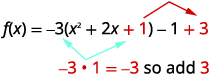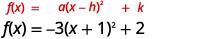# 9.8：使用变换绘制二次函数图

•• OpenStax
• OpenStax
$$\newcommand{\vecs}{\overset { \rightharpoonup} {\mathbf{#1}} }$$ $$\newcommand{\vecd}{\overset{-\!-\!\rightharpoonup}{\vphantom{a}\smash {#1}}}$$$$\newcommand{\id}{\mathrm{id}}$$ $$\newcommand{\Span}{\mathrm{span}}$$ $$\newcommand{\kernel}{\mathrm{null}\,}$$ $$\newcommand{\range}{\mathrm{range}\,}$$ $$\newcommand{\RealPart}{\mathrm{Re}}$$ $$\newcommand{\ImaginaryPart}{\mathrm{Im}}$$ $$\newcommand{\Argument}{\mathrm{Arg}}$$ $$\newcommand{\norm}{\| #1 \|}$$ $$\newcommand{\inner}{\langle #1, #2 \rangle}$$ $$\newcommand{\Span}{\mathrm{span}}$$ $$\newcommand{\id}{\mathrm{id}}$$ $$\newcommand{\Span}{\mathrm{span}}$$ $$\newcommand{\kernel}{\mathrm{null}\,}$$ $$\newcommand{\range}{\mathrm{range}\,}$$ $$\newcommand{\RealPart}{\mathrm{Re}}$$ $$\newcommand{\ImaginaryPart}{\mathrm{Im}}$$ $$\newcommand{\Argument}{\mathrm{Arg}}$$ $$\newcommand{\norm}{\| #1 \|}$$ $$\newcommand{\inner}{\langle #1, #2 \rangle}$$ $$\newcommand{\Span}{\mathrm{span}}$$$$\newcommand{\AA}{\unicode[.8,0]{x212B}}$$

##### 学习目标

• 图形形式的二次函数$$f(x)=x^{2}+k$$
• 图形形式的二次函数$$f(x)=(x−h)^{2}$$
• 图形形式的二次函数$$f(x)=ax^{2}$$
• 使用变换绘制二次函数的图形
• 从其图形中找到二次函数

1. $$f(x)=x^{2}$$通过绘制点来绘制函数图表。
如果你错过了这个问题，请查看示例 3.54。
2. 完全考虑因素:$$y^{2}−14y+49$$.
如果您错过了此问题，请查看示例 6.24。
3. 完全考虑因素:$$2x^{2}−16x+32$$.
如果您错过了此问题，请查看示例 6.26。

## 绘制表格的二次函数$$f(x)=x^{2}+k$$

##### 示例$$\PageIndex{1}$$

$$g(x)=x^{2}+2$$图形与单位的图形相同，$$f(x)=x^{2}$$$$2$$单位向上移动。

##### 练习$$\PageIndex{1}$$
1. 在同一个直角坐标系$$h(x)=x^{2}-1$$上绘制图$$f(x)=x^{2}, g(x)=x^{2}+1,$$形。
2. 描述在函数中添加常量对基本抛物线有什么影响。

b. 的图形与单位的$$g(x)=x^{2}+1$$图形相同，$$f(x)=x^{2}$$$$1$$单位向上移动。 的图形$$h(x)=x^{2}−1$$与图形相同，$$f(x)=x^{2}$$$$1$$单位向下移动。

##### 练习$$\PageIndex{2}$$
1. 在同一个直角坐标系$$h(x)=x^{2}-6$$上绘制图$$f(x)=x^{2}, g(x)=x^{2}+6,$$形。
2. 描述在函数中添加常量对基本抛物线有什么影响。

b. 的图形与单位的$$h(x)=x^{2}+6$$图形相同，$$f(x)=x^{2}$$$$6$$单位向上移动。 的图形$$h(x)=x^{2}-6$$与单位的图形相同，$$f(x)=x^{2}$$$$6$$单位向下移动。

### $$f(x)=x^{2}+k$$使用垂直移位绘制形状的二次函数

• 如果是$$k>0$$，则将抛物线垂直向上移动$$k$$单位。
• 如果为$$k<0$$，则将抛物线垂直向下移动$$|k|$$单位。

$$f(x)=x^{2}$$快速练习素描可能会有所帮助。 我们知道这些值，可以从那里绘制图表。

##### 示例$$\PageIndex{2}$$

$$f(x)=x^{2}−3$$使用垂直移位绘制图表。

 我们首先在网格$$f(x)=x^{2}$$上画出图形。确定$$k$$。$$f(x)=x^{2}$$向下移动图表$$3$$。##### 练习$$\PageIndex{3}$$

$$f(x)=x^{2}−5$$使用垂直移位绘制图表。

##### 练习$$\PageIndex{4}$$

$$f(x)=x^{2}+7$$使用垂直移位绘制图表。

## 绘制表格的二次函数$$f(x)=(x-h)^{2}$$

##### 练习$$\PageIndex{5}$$
1. 在同一个直角坐标系$$h(x)=(x-2)^{2}$$上绘制图$$f(x)=x^{2}, g(x)=(x+2)^{2},$$形。
2. 描述在函数中添加常量对基本抛物线有什么影响。

b. 的$$g(x)=(x+2)^{2}$$图形与$$2$$单位向左移动$$f(x)=x^{2}$$的图形相同。 的$$h(x)=(x−2)^{2}$$图形与单位的图形相同，$$f(x)=x^{2}$$$$2$$单位向右移动。

##### 练习$$\PageIndex{6}$$
1. 在同一个直角坐标系$$h(x)=x^{2}-5$$上绘制图$$f(x)=x^{2}, g(x)=x^{2}+5,$$形。
2. 描述在函数中添加常量对基本抛物线有什么影响。

b. 的$$g(x)=(x+5)^{2}$$图形与$$5$$单位向左移动$$f(x)=x^{2}$$的图形相同。 的图形$$h(x)=(x-5)^{2}$$与图形相同，$$f(x)=x^{2}$$$$5$$单位向右移动。

### $$f(x)=(x-h)^{2}$$使用水平移位绘制表格的二次函数

• 如果是$$h>0$$，则将抛物线水平向左移动$$h$$单位。
• 如果是$$h<0$$，则将抛物线水平向右移动$$|h|$$单位。

##### 示例$$\PageIndex{4}$$

$$f(x)=(x−6)^{2}$$使用水平移位绘制图表。

 我们首先在网格$$f(x)=x^{2}$$上画出图形。确定$$h$$。将图表移动$$f(x)=x^{2}$$到右边的$$6$$单位。##### 练习$$\PageIndex{7}$$

$$f(x)=(x−4)^{2}$$使用水平移位绘制图表。

##### 练习$$\PageIndex{8}$$

$$f(x)=(x+6)^{2}$$使用水平移位绘制图表。

##### 示例$$\PageIndex{5}$$

$$f(x)=(x+1)^{2}-2$$使用转换绘制图表。

$$h$$常数给我们一个水平移动，$$k$$给我们一个垂直移动。

##### 练习$$\PageIndex{9}$$

$$f(x)=(x+2)^{2}-3$$使用转换绘制图表。

##### 练习$$\PageIndex{10}$$

$$f(x)=(x-3)^{2}+1$$使用转换绘制图表。

## 绘制表格的二次函数$$f(x)=ax^{2}$$

### 形态的二次函数图$$f(x)=ax^{2}$$

• 如果$$0<|a|<1$$，的图形$$f(x)=ax^{2}$$将比的图形 “宽”$$f(x)=x^{2}$$
• 如果$$|a|>1$$，的图表$$f(x)=ax^{2}$$将比的图形 “更薄”$$f(x)=x^{2}$$

## 使用变换绘制二次函数图

##### 示例$$\PageIndex{7}$$将$$x$$项与常量分开。将系数分解为$$x^{2}, -3$$。准备完成方块。取一半$$2$$然后将其平整以完成正方形$$(\frac{1}{2}\cdot 2)^{2}=1$$ 常量$$1$$补齐圆括号中的正方形，但圆括号乘以$$-3$$。 所以我们真的在补充$$-3$$。 然后，我们必须$$3$$添加而不是更改函数的值。将三项式重写为正方形并减去常量。该函数现在采用表$$f(x)=a(x-h)^{2}+k$$单。##### 练习$$\PageIndex{13}$$

$$f(x)=-4(x+1)^{2}+5$$

##### 练习$$\PageIndex{14}$$

$$f(x)=2(x-2)^{2}-5$$

##### 示例$$\PageIndex{8}$$将$$x$$项与常量分开。取一半$$6$$然后将其平整以完成正方形。 $$(\frac{1}{2}\cdot 6)^{2}=9$$ 我们$$9$$加减都是$$9$$为了不改变函数的值。将三项式重写为正方形并减去常量。该函数现在采用表$$f(x)=(x-h)^{2}+k$$单。### 使用变换绘制二次函数的图形

1. 通过填写方块来重写$$f(x)=a(x-h)^{2}+k$$表单中的函数。
2. 使用转换绘制函数图表。
##### 示例$$\PageIndex{9}$$将$$x$$项与常量分开。我们需要将的系数$$x^{2}$$设为一。 我们$$-2$$从$$x$$-terms中考虑因素。取一半$$2$$然后将其平整以完成正方形。 $$(\frac{1}{2}\cdot 2)^{2}=1$$ 我们在圆括号中加上方形$$1$$以完成方块，但圆括号乘以$$-2$$。 所以我们真的在补充$$-2$$。 为了不更改我们添加的函数的值$$2$$。将三项式重写为正方形并减去常量。该函数现在采用表$$f(x)=a(x-h)^{2}+k$$单。### $$f(x)=a(x-h)^{2}+k$$使用属性绘制表单中的二次函数

1. 重写函数表$$f(x)=a(x-h)^{2}+k$$单。
2. 确定抛物线是向上打开$$a>0$$，还是向下打开$$a<0$$
3. 找到对称轴，$$x=h$$
4. 找到顶点，$$(h,k$$
5. 找到$$y$$截距。 在对称轴上找到$$y$$与-截距对称的点。
6. 找到$$x$$-截图。
7. 绘制抛物线图。
##### 示例$$\PageIndex{10}$$
1. $$f(x)=2 x^{2}+4 x+5$$$$f(x)=a(x-h)^{2}+k$$表单中重写
2. 使用属性绘制函数图表

 通过填写方块来重写$$f(x)=a(x-h)^{2}+k$$表单中的函数。 $$f(x)=2 x^{2}+4 x+5$$ $$f(x)=2\left(x^{2}+2 x\right)+5$$ $$f(x)=2\left(x^{2}+2 x+1\right)+5-2$$ $$f(x)=2(x+1)^{2}+3$$ 识别常量$$a, h, k$$。 $$a=2 h=-1 k=3$$ 因为$$a=2$$，抛物线向上打开。对称轴是$$x=h$$。 对称轴是$$x=-1$$。 顶点是$$(h,k)$$。 顶点是$$(-1,3)$$。 通过查找找到$$y$$-截距$$f(0)$$。 $$f(0)=2 \cdot 0^{2}+4 \cdot 0+5$$ $$f(0)=5$$ $$y$$-截距$$(0,5)$$ 找到与对称轴$$(0,5)$$对称的点。 $$(-2,5)$$ 找到$$x$$-截图。 判别值为负，因此没有$$x$$-intercepts。 绘制抛物线图。##### 练习$$\PageIndex{19}$$
1. $$f(x)=3 x^{2}-6 x+5$$$$f(x)=a(x-h)^{2}+k$$表单中重写
2. 使用属性绘制函数图表

1. $$f(x)=3(x-1)^{2}+2$$

2.图 9.7.66
##### 练习$$\PageIndex{20}$$
1. $$f(x)=-2 x^{2}+8 x-7$$$$f(x)=a(x-h)^{2}+k$$表单中重写
2. 使用属性绘制函数图表

1. $$f(x)=-2(x-2)^{2}+1$$

2.图 9.7.67

## 从其图形中找到二次函数

##### 示例$$\PageIndex{11}$$

$$f(x)=a(x-(-2))^{2}-1$$

$$7=a(0+2)^{2}-1$$

$$\begin{array}{l}{7=4 a-1} \\ {8=4 a} \\ {2=a}\end{array}$$

$$f(x)=a(x-h)^{2}+k$$

$$h=-2, k=-1$$和中替换$$a=2$$

$$f(x)=2(x+2)^{2}-1$$

##### 练习$$\PageIndex{21}$$

$$f(x)=(x-3)^{2}-4$$

##### 练习$$\PageIndex{22}$$

$$f(x)=(x+3)^{2}-1$$

## 关键概念

• $$f(x)=x^{2}+k$$使用垂直移位绘制形状的二次函数
• 的图表会$$f(x)=x^{2}+k$$移动$$f(x)=x^{2}$$垂直$$k$$单位的图形。
• 如果是$$k>0$$，则将抛物线垂直向上移动$$k$$单位。
• 如果为$$k<0$$，则将抛物线垂直向下移动$$|k|$$单位。
• $$f(x)=(x−h)^{2}$$使用水平偏移绘制形状的二次函数
• 的图表会$$f(x)=(x−h)^{2}$$移动$$f(x)=x^{2}$$水平$$h$$单位的图形。
• 如果是$$h>0$$，则将抛物线水平向左移动$$h$$单位。
• 如果是$$h<0$$，则将抛物线水平向右移动$$|h|$$单位。
• 形式为二次函数的图形$$f(x)=ax^{2}$$
• 函数$$a$$中的系数$$f(x)=x^{2}$$通过拉伸或压缩来$$f(x)=ax^{2}$$影响图形。
如果$$0<|a|<1$$，则的图形$$f(x)=ax^{2}$$将比的图形 “宽”$$f(x)=x^{2}$$
如果$$|a|>1$$，则的图表$$f(x)=ax^{2}$$将比的图形 “更薄”$$f(x)=x^{2}$$
• 如何使用变换绘制二次函数的图形
1. 通过填写方块来重写$$f(x)=a(x−h)^{2}+k$$表单中的函数。
2. 使用转换绘制函数图表。
• $$f(x)=a(x−h)^{2}+k$$使用属性以顶点形式绘制二次函数的图形
1. $$f(x)=a(x−h)^{2}+k$$表单重写函数。
2. 确定抛物线是向上打开$$a>0$$，还是向下打开$$a<0$$
3. 找到对称轴，$$x=h$$
4. 找到顶点，$$(h,k)$$
5. 找到$$y$$截距。 在对称轴上找到$$y$$与-截距对称的点。
6. 如果可能的话，找到$$x$$-intercepts。
7. 绘制抛物线图。